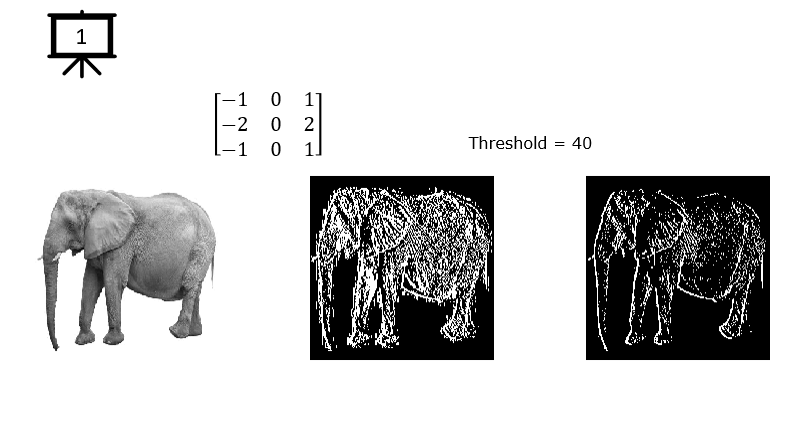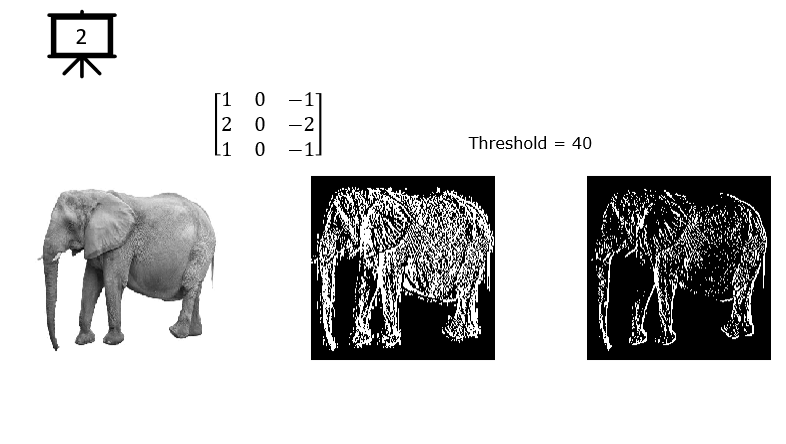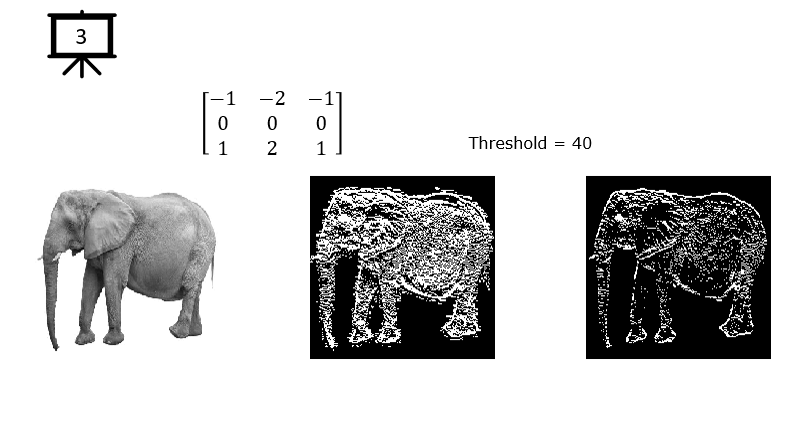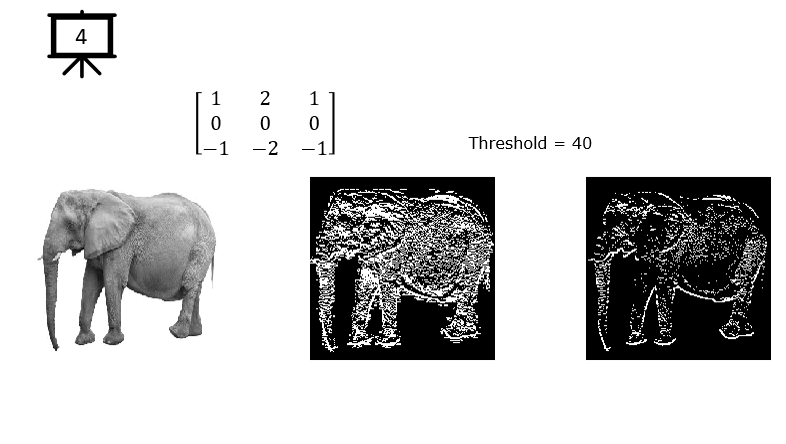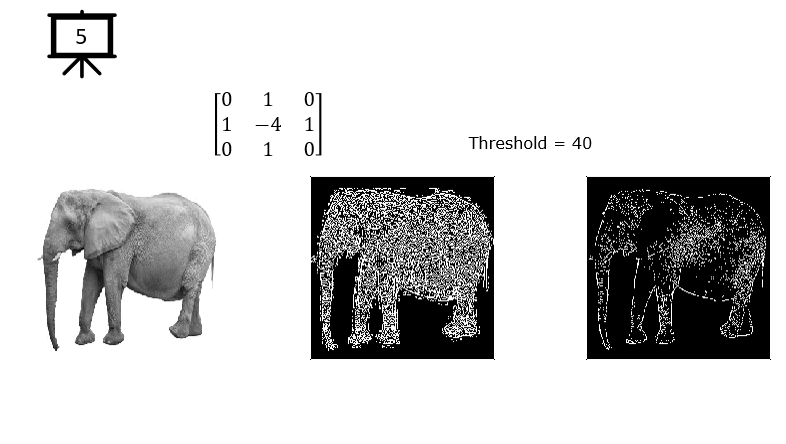2D Convolution - Edge Detection   Convolution would be the one of the most important operations in engineering math. If you don't have any prior study and understanding on how Convolution works, it would not be easy to make sense out of it. For those who is not familiar with Convolution, I would suggest you to go through my descriptive note here.   Convolution would be the one of the most important operations in engineering math. If you don't have any prior study and understanding on how Convolution works, it would not be easy to make sense out of it. For those who is not familiar with Convolution, I would suggest you to go through my descriptive note here.   The example in this page is to show how the convolution works for 2D data(i.e, 2D array data like an image). This 2D convolution has long been used for the purpose of various image processing (especially for edge detection), but it has become very popular and important concept since it is used by a famous neural network/machine learning algorithm called CNN.   I assume that you have clear understanding on 2D convolution from previous examples in term of calcuation process. This example is designed to give you some intuitive understanding on the meaning of several famous kernel (also called 'filter').

 Followings are the code that I wrote in Octave to creates all the plots shown in this page. You may copy these code and play with these codes. Change variables and try yourself until you get your own intuitive understanding.   < Code 1 >   img = imread ("img_Shape_01.png");   img = img(:,:,1); flt = [-1 0 1; ...        -2 0 2; ...        -1 0 1];    flt = [1 0 -1; ...        2 0 -2; ...        1 0 -1];   flt = [-1 -2 -1; ...         0  0  0; ...         1  2  1];     flt = [ 1  2  1; ...         0  0  0; ...        -1 -2 -1];    flt = [ 0  1  0; ...         1  -4  1; ...         0  1  0];   imgConv = conv2(img, flt); imgConvTh = imgConv;   th = 40; imgConvTh(imgConvTh < th) = 0;   %imgConv = edge(img, "Canny");    %imgConv = edge(img, "Sobel");     hFig = figure(1,'Position',[300 300 950 540]);   subplot(1,3,1); imshow(img);   subplot(1,3,2); imshow(imgConv);   subplot(1,3,3); imshow(imgConvTh);